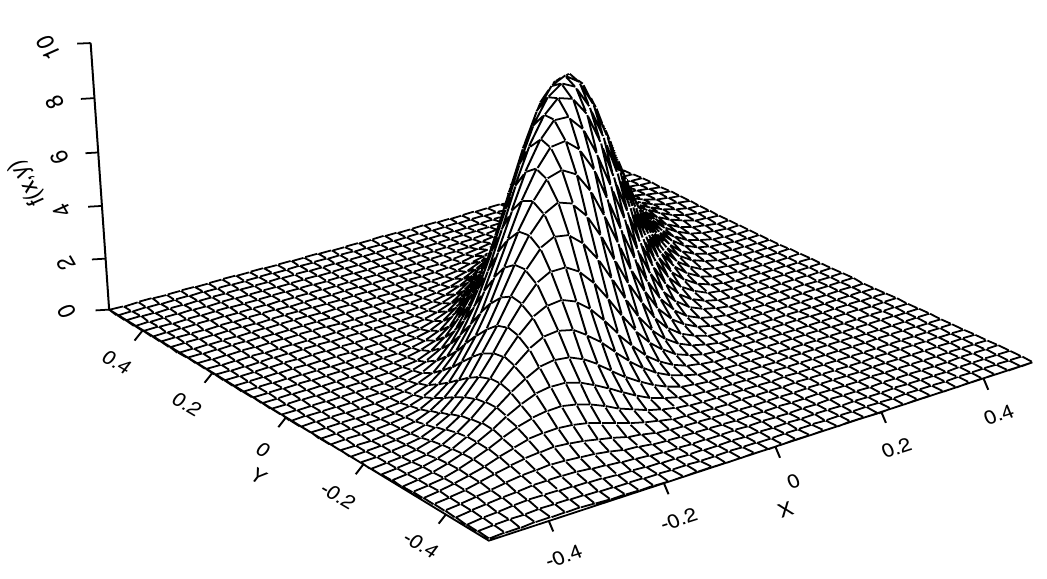# A review of random vectors

## Instructions:

Use the left and right arrow keys to navigate the presentation forward and backward respectively. You can also use the arrows at the bottom right of the screen to navigate with a mouse.

FAIR USE ACT DISCLAIMER:
This site is for educational purposes only. This website may contain copyrighted material, the use of which has not been specifically authorized by the copyright holders. The material is made available on this website as a way to advance teaching, and copyright-protected materials are used to the extent necessary to make this class function in a distance learning environment. The Fair Use Copyright Disclaimer is under section 107 of the Copyright Act of 1976, allowance is made for “fair use” for purposes such as criticism, comment, news reporting, teaching, scholarship, education and research.

## Outline

• The following topics will be covered in this lecture:
• Multiple random variables
• CDF and PDF of multiple variables
• Marginals
• The ensemble matrix
• The expected value
• The ensemble mean

## Introducing multiple random variables

• We will now introduce the basic tools of statistics and probability theory for multivariate analysis;

• we will be studying the relations between $$N_x>1$$ total RVs that will often covary together in their conditional probabilities.
• Some notions like the expected / center of mass will translate directly over linear combinations of RVs.

• Recall, for RVs $$X,Y$$ and a constant scalars $$a,b$$ we have $\mathbb{E}\left[ a X + b Y\right] = a \mathbb{E}\left[X\right] + b \mathbb{E}\left[Y\right]$ by the linearity of the expectation.

• Recall the vector notation $$\pmb{x} \in \mathbb{R}^{N_x}$$, matrix notation $$\mathbf{A} \in \mathbb{R}^{N_x \times N_x}$$, and matrix-slice notation $$\mathbf{A}^j \in \mathbb{R}^{N_x}$$ where

\begin{align} \pmb{x} := \begin{pmatrix} x_1 \\ \vdots \\ x_{N_x} \end{pmatrix} & & \mathbf{A} := \begin{pmatrix} a_{1,1} & \cdots & a_{1, N_x} \\ \vdots & \ddots & \vdots \\ a_{N_x,1} & \cdots & a_{N_x, N_x} \end{pmatrix} & & \mathbf{A}^j := \begin{pmatrix} a_{1,j} \\ \vdots \\ a_{N_x, j} \end{pmatrix} \end{align}

• The linearity of the expectation then extends to random vectors $$\pmb{x}, \pmb{y}$$ and constant matrices $$\mathbf{A},\mathbf{B}$$, we can write $\mathbb{E}\left[\mathbf{A}\pmb{x} + \mathbf{B}\pmb{y} \right] = \mathbf{A}\mathbb{E}\left[ \pmb{x}\right] + \mathbf{B}\mathbb{E}\left[\pmb{y}\right].$

• While the concept of the center of mass has a direct generalization to vectors, we will need to make some additional considerations when we measure the spread of RVs and how they relate to others.

• The extension of the second centered moment has the generalization to the rotational inertia tensor.

## The cumulative distribution function

• We will begin our consideration in $$N_x=2$$ dimensions, as all properties described in the following will extend (with minor modifications) to arbitrarily large but finite $$N_x$$.

• Let the random vector $$\pmb{X}$$ be defined as

\begin{align} \pmb{X} = \begin{pmatrix} X_1 \\ X_2 \end{pmatrix} \end{align} where each of the above components $$X_i$$ is a RV.

• We can define the cumulative distribution function in a similar way to the definition in one variable.

• Let $$x_1,x_2$$ be two fixed real values forming a constant vector as

$\pmb{x} = \begin{pmatrix} x_1 \\ x_2\end{pmatrix}.$

• Define the comparison operator between two vectors $$\pmb{y}, \pmb{x}$$ as

$\pmb{y} \leq \pmb{x} \Leftrightarrow y_i \leq x_i \text{ for each and every }i$

Multivariate cumulative distribution function
The cumulative distribution function $$P$$, describing the probability of realizations of $$\pmb{X}$$, is defined $P(\pmb{x}) = \mathcal{P}(\pmb{X}\leq \pmb{x} ) = \mathcal{P}(X_i \leq x_i \quad \forall i=1,\cdots,N_x).$

### The joint probability density function

• Recall that the CDF

\begin{align} P:\mathbb{R}^2 & \rightarrow [0,1] \\ \pmb{x} & \rightarrow \mathcal{P}(\pmb{X}\leq \pmb{x}) \end{align} is a function of the variables $$(x_1,x_2)$$.

• Suppose then that $$P$$ has continuous second partial derivatives in $$\partial_{x_1} \partial_{x_2}P = \partial_{x_2}\partial_{x_1}P$$;

• this implies we can arbitrarily exchange the order of differentiation or integration.
Multivariate probability density function
Let $$P\in \mathcal{C}^2\left(\mathbb{R}^2\right)$$ , then the probability density function $$p$$ is defined as \begin{align} p:\mathbb{R}^2 & \rightarrow \mathbb{R}\\ \pmb{x} &\rightarrow \partial_{x_1}\partial_{x_2}P(\pmb{x}) \end{align}
• In the above definition, we have constructed the density function in the same way as in one variable;

• specifically, in the case where $$p$$ itself is differentiable, we have defined the CDF as the anti-derivative of the density:

\begin{align} P(\pmb{x}) = \int_{-\infty}^{x_1} \int_{-\infty}^{x_2} p(s_1, s_2) \mathrm{d}s_1 \mathrm{d}s_2. \end{align}

### The joint probability density function

• Recall that the relationship between the CDF and the PDF as

\begin{align} P(\pmb{x}) = \int_{-\infty}^{x_1} \int_{-\infty}^{x_2} p(s_1, s_2) \mathrm{d}s_1 \mathrm{d}s_2; \end{align}

• this implies that

\begin{align} P(- \infty < \pmb{X} < \infty) = \int_{-\infty}^{\infty} \int_{-\infty}^{\infty} p(s_1, s_2) \mathrm{d}s_1 \mathrm{d}s_2 = 1 \end{align}

• If we define this over $$N_x\geq 2$$ variables, all of the above extends identically when $$P$$ has derivatives defined in arbitrary arrangements of the $$N_x$$-th partial derivatives in each $$\partial_{x_i}$$.

• Specifically, this requires that, for all permutations $$\psi:(1, \cdots, N_x) \rightarrow (\psi(1), \cdots ,\psi(N_x))$$,

\begin{align} \partial_{x_1} \cdots \partial_{x_{N_x}}P = \partial_{x_{\psi(1)}} \cdots \partial_{x_{\psi(N_x)}}P \end{align}

• We then construct $$p$$ as the $$N_x$$-th partial derivative of $$P$$ in all univariate partial derivatives.
• Using the integral relationship, we can similarly show

\begin{align} P(\pmb{x}) = \int_{-\infty}^{x_1} \cdots \int_{-\infty}^{x_{N_x}} p(s_1, \cdots, s_{N_x}) \mathrm{d}s_1\cdots \mathrm{d}s_{N_x}. \end{align}

### The joint probability density function

• Particularly, we will again view the density function like the curve of the univariate case, but for two variables we see this as a surface above the $$x_1,x_2$$ plane.Courtesy of: F.M. Dekking, et al. A Modern Introduction to Probability and Statistics. Springer Science & Business Media, 2005.

• To the right, we see the multivariate Gaussian bell surface that defines the multivariate normal distribution in two variables.
• In one variable, the probability $$\mathcal{P}(X_1 \leq x_1)$$ was associated to the area under the curve, computed by the integral of the density.